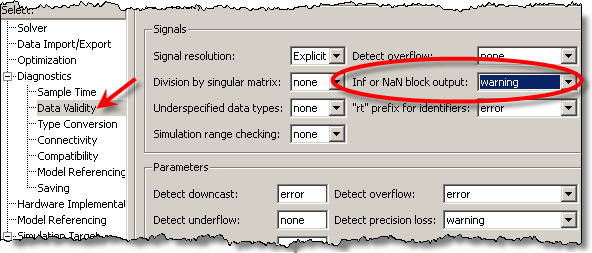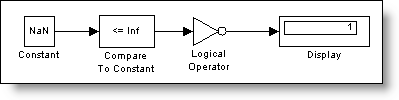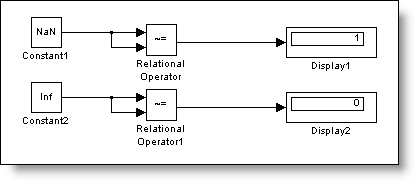# How do I test for NaN in my model?

This week I want to pose a brainteaser that my colleague
Jeff had to solve this week.  Imagine you need to detect the condition where a
signal value might become NaN (Not A Number).  How do you

What is a NaN?

NaN means Not-A-Number
In general math terms, this is the result for special cases, which are undefined.
In general computing terms, operations return NaN for invalid inputs.  For
example:

>> Inf*0

ans =

NaN

>> 0/0

ans =

NaN

>> Inf/Inf

ans =

NaN

>> Inf-Inf

ans =

NaN

If you are writing code and need to protect against invalid
inputs, detecting this could be important.

ISNAN?

In MATLAB we have the function ISNAN
ISNAN is a MATLAB function, which we could call from Simulink.

>> x = 0/0

x =

NaN

>> isnan(x)

ans =

1

In some ways, that feels like cheating.  If we were
developing this algorithm to run on an embedded system, MATLAB will not be
available.

First try, Diagnostics

The first idea I had was to look at the diagnostics.  I
remembered seeing a warning about NaNs once during a simulation, so there might
be a way to turn on a diagnostic to detect this.We found the diagnostic in the Data Validity page of the
Configuration Parameters.  This diagnostic will check for Inf or NaN signals in
a block output, but that doesn’t help if we want the model to do something
reactive with that information.  This diagnostic is purely informative.

NaN has a specific bit pattern

What about testing the bit pattern?  Using MATLAB I can see
what that pattern is in HEX:

>> format hex

>> NaN

ans =

fff8000000000000

We did try the bit operations, but ran into some
difficulties.  NaN is a floating-point number, and all the bit processing
blocks are built for working with integers.  In this case, working with a floating-point
number would require some contortions to get the bit pattern.  Once we had it,
we could use the other Bitwise Operator block to test for the pattern we were
looking for, but we didn’t get that working before we had another realization.

Comparison with NaN is different from all other numbers

That was the “Eureka!” moment: NaN has special properties!  Math
operations performed with NaN result in a NaN  (x+NaN = NaN).  Comparisons to a
NaN return false.  For example:

>> NaN<1

ans =

0

>> NaN<=Inf

ans =

0

Comparison with Inf is pretty interesting because we couldn’t
think of any other numbers that would return FALSE for X<=Inf, except NaN.  Even if X=Inf, this should return TRUE.  In Simulink, the test
model looked like this:Using the logical operation, NOT, we can pass any signal
through these blocks and make our own ISNAN block.  Another interesting
property of NaN is that it is the ONLY number not equal to itself.  An
alternative model, arguably more streamlined, uses just the Relational Operator
to test the NOT Equals condition and compares the signal to itself.All numbers EXCEPT NaN should return FALSE for this test.
We double-checked it by sending infinity through the same construct.